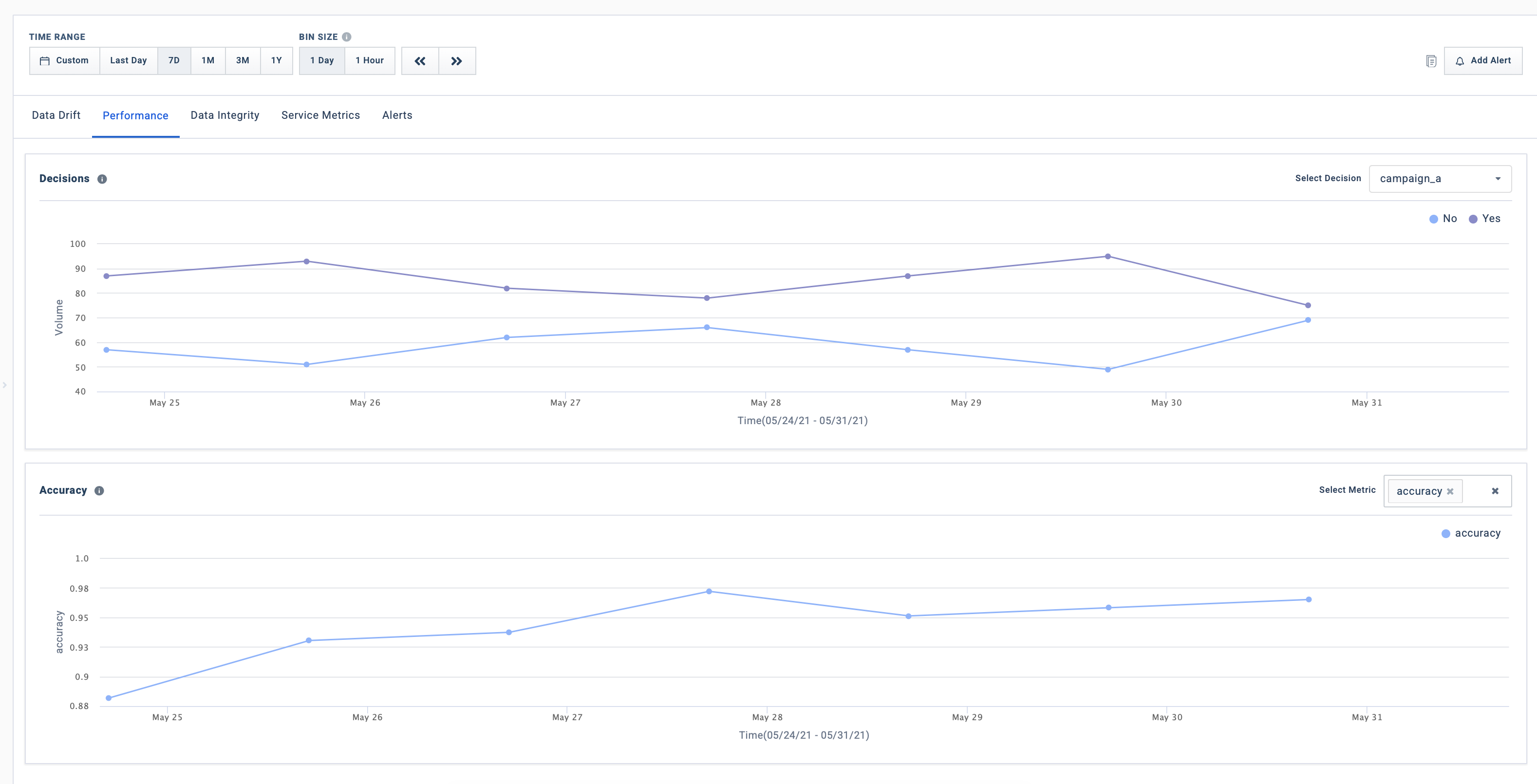# Performance Tracking

Platform Guide

## What is being tracked?• Decisions - The post-prediction business decisions made as a result of the model output. Decisions are calculated before client.publish_event() (they're not inferred by Fiddler). For binary classification models, a decision is usually determined using a threshold. For multi-class classification models, it's usually determined using the argmax value of the model outputs.

• Performance metrics

Binary ClassificationAccuracy(TP + TN) / (TP + TN + FP + FN)
Binary ClassificationTrue Positive Rate/RecallTP / (TP + FN)
Binary ClassificationFalse Positive RateFP / (FP + TN)
Binary ClassificationPrecisionTP / (TP + FP)
Binary ClassificationF1 Score2 * ( Precision * Recall ) / ( Precision + Recall )
Binary ClassificationAUROCArea Under the Receiver Operating Characteristic (ROC) curve, which plots the true positive rate against the false positive rate
Binary ClassificationBinary Cross EntropyMeasures the difference between the predicted probability distribution and the true distribution
Binary ClassificationGeometric MeanSquare Root of ( Precision * Recall )
Binary ClassificationCalibrated ThresholdA threshold that balances precision and recall at a particular operating point
Binary ClassificationData CountThe number of events where target and output are both not NULL. This will be used as the denominator when calculating accuracy.
Binary ClassificationExpected Calibration ErrorMeasures the difference between predicted probabilities and empirical probabilities
Multi ClassificationAccuracy(Number of correctly classified samples) / ( Data Count ). Data Count refers to the number of events where the target and output are both not NULL
Multi ClassificationLog LossMeasures the difference between the predicted probability distribution and the true distribution, in a logarithmic scale
RegressionCoefficient of determination (R-squared)Measures the proportion of variance in the dependent variable that is explained by the independent variables
RegressionMean Squared Error (MSE)Average of the squared differences between the predicted and true values
RegressionMean Absolute Error (MAE)Average of the absolute differences between the predicted and true values
RegressionMean Absolute Percentage Error (MAPE)Average of the absolute percentage differences between the predicted and true values
RegressionWeighted Mean Absolute Percentage Error (WMAPE)The weighted average of the absolute percentage differences between the predicted and true values
RankingMean Average Precision (MAP)—for binary relevance ranking onlyMeasures the average precision of the relevant items in the top-k results
RankingNormalized Discounted Cumulative Gain (NDCG)Measures the quality of the ranking of the retrieved items, by discounting the relevance scores of items at lower ranks

## Why is it being tracked?

• Model performance tells us how well a model is doing on its task. A poorly performing model can have significant business implications.
• The volume of decisions made on the basis of the predictions give visibility into the business impact of the model.

## What steps should I take based on this information?

• For decisions, if there is an increase or decrease in approvals, we can cross-check with the average prediction and prediction drift trendlines on the Data Drift Tab. In general, the average prediction value should increase with an increase in the number of approvals, and vice-versa.
• For changes in model performance—again, the best way to cross-verify the results is by checking the Data Drift Tab ). Once you confirm that the performance issue is not due to the data, you need to assess if the change in performance is due to temporary factors, or due to longer-lasting issues.
• You can check if there are any lightweight changes you can make to help recover performance—for example, you could try modifying the decision threshold.
• Retraining the model with the latest data and redeploying it is usually the solution that yields the best results, although it may be time-consuming and expensive.

Reference